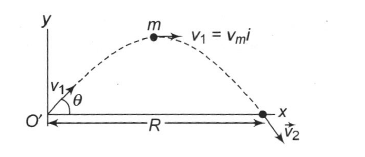A projectile of mass m is launched with an initial velocity vi→ making an angle θ with the horizontal as shown in figure. The projectile moves in the gravitational field of the Earth. The angular momentum of the projectile about the origin when it is at the highest point of its trajectory is

# A projectile of mass m is launched with an initial velocity $\stackrel{\to }{{\mathrm{v}}_{\mathrm{i}}}$ making an angle $\mathrm{\theta }$ with the horizontal as shown in figure. The projectile moves in the gravitational field of the Earth. The angular momentum of the projectile about the origin when it is at the highest point of its trajectory is1. A

2. B

3. C

4. D

Register to Get Free Mock Test and Study Material

+91

Verify OTP Code (required)

### Solution:

At the highest point of the trajectory,

The angular momentum is then

=

=Register to Get Free Mock Test and Study Material

+91

Verify OTP Code (required)